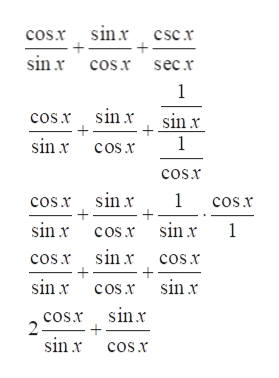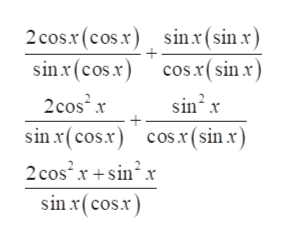# Verify the folowing equation is an identity with work showncos(x)/sin(x)+sin(x)/cos(x)+csc(x)/sec(x)=(sec(x)+cos(x))/sin(x)

Question
17 views

Verify the folowing equation is an identity with work shown

cos(x)/sin(x)+sin(x)/cos(x)+csc(x)/sec(x)=(sec(x)+cos(x))/sin(x)

check_circle

Step 1

Given equation is

Step 2

To verify the identity , start from left hand side of the equation .

Use trigonometric ratios csc(x)= 1/sinx and sec(x)= 1/cos(x)help_outlineImage Transcriptionclosecos.r sinr csc r + sin x cos.x secr 1 cos.x sinx sin x + 1 sin x cosx cos.x cos x sinx 1 cos.x sin x cos.r sin x 1 cos.r sin x cos.x sinx cosr sin x cos.r sinr 2 sinxcosx fullscreen
Step 3

Take LCD sinx cosx and si...help_outlineImage Transcriptionclose2cos.r(cos.x) sinx(sin x) sin x (cos.x) cos.x(sin x) 2cos2 x sin2 x sin r(cosx) cos.x(sin x) 2 cos2x+sinx sin x(cos.x) fullscreen

### Want to see the full answer?

See Solution

#### Want to see this answer and more?

Solutions are written by subject experts who are available 24/7. Questions are typically answered within 1 hour.*

See Solution
*Response times may vary by subject and question.
Tagged in